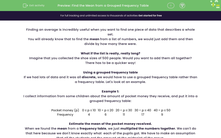# Find the Mean from a Grouped Frequency Table

In this worksheet, students will find the mean from a grouped frequency table.Key stage:  KS 4

Year:  GCSE

GCSE Subjects:   Maths

GCSE Boards:   AQA, Eduqas, Pearson Edexcel, OCR,

Curriculum topic:   Statistics

Curriculum subtopic:   Statistics Analysing Data

Difficulty level:#### Worksheet Overview

Finding an average is incredibly useful when you want to find one piece of data that describes a whole set.

You will already know that to find the mean from a list of numbers, we would just add them and then divide by how many there were.

What if the list is really, really long?

Imagine that you collected the shoe sizes of 500 people. Would you want to add them all together?

There has to be a quicker way!

Using a grouped frequency table

If we had lots of data and it was all discrete, we would have to use a grouped frequency table rather than a frequency table. Let's look at an example.

Example 1:

I collect information from some children about the amount of pocket money they receive, and put it into a grouped frequency table:

 Pocket money (p) 0 ≤ p ≤ 10 10 < p ≤ 20 20 < p ≤ 30 30 < p ≤ 40 40 < p ≤ 50 Frequency 4 6 11 17 9

Estimate the mean of the pocket money received.

When we found the mean from a frequency table, we just multiplied the numbers together. We can't do that here because we don't know exactly what  each of the pupils got. We have to make an assumption that all the students got the amount at the midpoint of the group.

 Pocket money (p) 0 ≤ p ≤ 10 10 < p ≤ 20 20 < p ≤ 30 30 < p ≤ 40 40 < p ≤ 50 Frequency 4 6 11 17 9 Midpoint 5 15 25 35 45

Now we can multiply together the midpoints and the frequency:

 Pocket Money (p) 0 ≤ p ≤ 10 10 < p ≤ 20 20 < p ≤ 30 30 < p ≤ 40 40 < p ≤ 50 Frequency 4 6 11 17 9 Midpoint 5 15 25 35 45 mp x f 20 90 275 595 405

Once we have this, we can work out an estimate of the total pocket money by adding the numbers in red. (1385 )

We now have one of the bits of information we need to find the mean (what they all add up to).

All we need now is to find out how many people were asked. We can get this by adding up all the numbers in the frequency row, which add up to  47.

To find the mean, all we now have to do is to divide one of these numbers by the other: 1385 ÷ 47 = £29.47

A key point

You will probably see the word estimate in the question. We are not finding the mean, we are estimating it.

When you have grouped data, we made the assumption earlier that everyone got the value in the middle, this means that we cannot be certain what the mean is, we can only estimate it.

To summarise:

Step 1: Find the midpoints.

Step 2: Multiply the midpoints and the frequencies together.

Step 3: Add all these new numbers up.

Step 4: Add up all the frequencies.

Step 5: Divide to find the mean.

Let's have a go at some questions now.

### What is EdPlace?

We're your National Curriculum aligned online education content provider helping each child succeed in English, maths and science from year 1 to GCSE. With an EdPlace account you’ll be able to track and measure progress, helping each child achieve their best. We build confidence and attainment by personalising each child’s learning at a level that suits them.

Get started